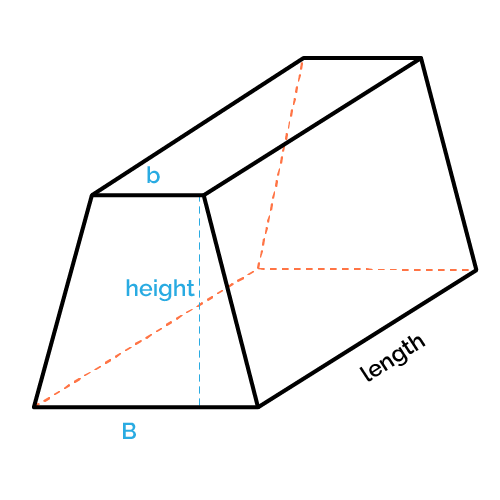# Volume of a Trapezoidal Prism Calculator

Created by Wei Bin Loo
Reviewed by Anna Szczepanek, PhD and Steven Wooding
Last updated: Jun 05, 2023

With this volume of a trapezoidal prism calculator, we aim to help you to calculate the volume of a trapezoidal prism. You can check out our trapezoid calculator and area of a trapezoid calculator to understand more about this topic.

We have written this article to help you understand what the volume of a trapezoidal prism is and how to find the volume of a trapezoidal prism. We will also demonstrate some examples to help you understand the trapezoidal prism volume formula.

## What is volume of a 3D object?

The volume of a 3D object is the amount of space occupied by the 3D object. The bigger the volume, the more space the object takes up. One way to measure volume is to measure the water the object displaces when it is put into water.

## How to find the volume of a trapezoidal prism?

To understand the formula for the volume of a trapezoidal prism, let's look at the example below:

• Length of short base (b): 5 m
• Length of long base (B): 5 m
• Length (ℓ): 5 m
• Height (h): 3 m
1. Determine the length of the short base (b).

The first step is to determine the length of the short base, b, of the trapezoidal prism. For our example, b is 5 m.

2. Determine the length of the long base (B).

The next step is to determine the length of the long base, B, of the trapezoidal prism. For our example, b is 5 m.

3. Calculate the length (ℓ).

Now you need to compute the length, , of the trapezoidal prism. The of this trapezoidal prism is 5 m.

4. Determine the height (h).

Also, the height, h, of the trapezoidal prism is 3 m.

5. Calculate the volume of a trapezoidal prism.

The last step is to calculate the volume of the trapezoidal prism using the formula:

((b + B) / 2) × h × ℓ

Thus, the volume of the trapezoidal prism is ((5 m + 5 m) / 2) × 5 m × 3 m = 75 m³.

## FAQ

### Can volume be negative?

No, volume cannot be negative. This is because zero and negative volume simply doesn't exist. An object cannot have zero or negative volume.

### What is the volume of a trapezoidal prism with all 1 m sides?

The volume of that trapezoidal prism will be 1 m³. You can calculate it by using this formula:

volume = ((short base length + long base length) / 2) × height × length.

### How can I calculate the volume of a trapezoidal prism?

You can calculate the volume of a trapezoidal prism in five steps:

1. Determine the length of the short base (b)

2. Determine the length of the long base (B)

3. Calculate the length (ℓ)

4. Determine the height (h)

5. Apply the trapezoidal prism volume formula:

((b + B) / 2) × h × ℓ

### What is lateral area for a 3D object?

The lateral area of a 3D object is the sum of the areas of all the sides of a 3D object besides the base and the top.

Wei Bin Loo
Height
m
Length
m
Long base (B)
m
Short base (b)
m
VolumePeople also viewed…

### Linear combination

Linear combination calculator helps you solve a system of equations with the method of combining linear equations.

### SAS triangle

You can calculate every quantity in a triangle just by giving us two sides and an angle: try our SAS triangle calculator!

### Significant figures

The significant figures calculator performs operations on sig figs and shows you a step-by-step solution!

### Steps to calories

Steps to calories calculator helps you to estimate the total amount to calories burned while walking.